📆 13 May 2022
📂 Gallery Type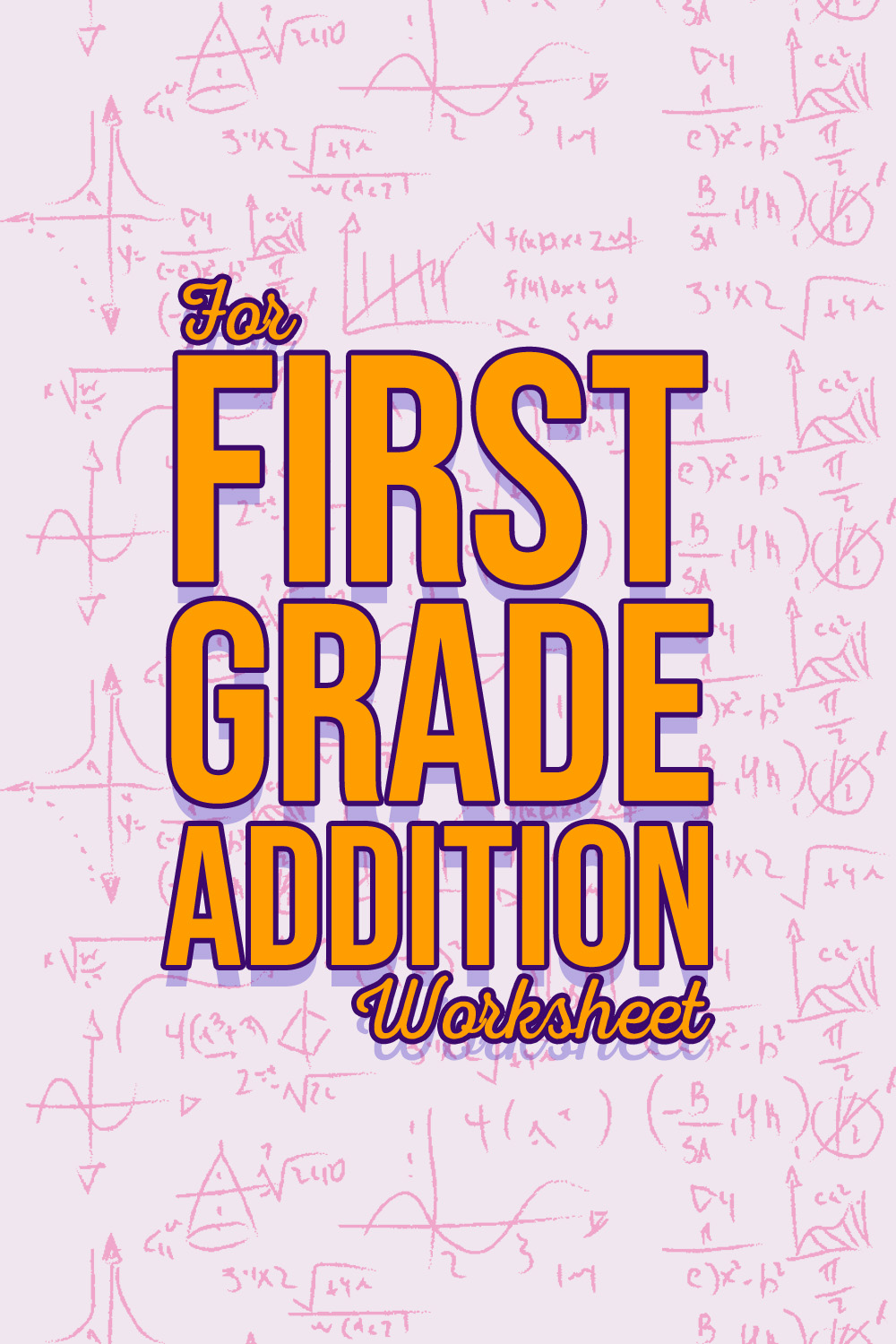12 Images of For First Grade Addition Worksheets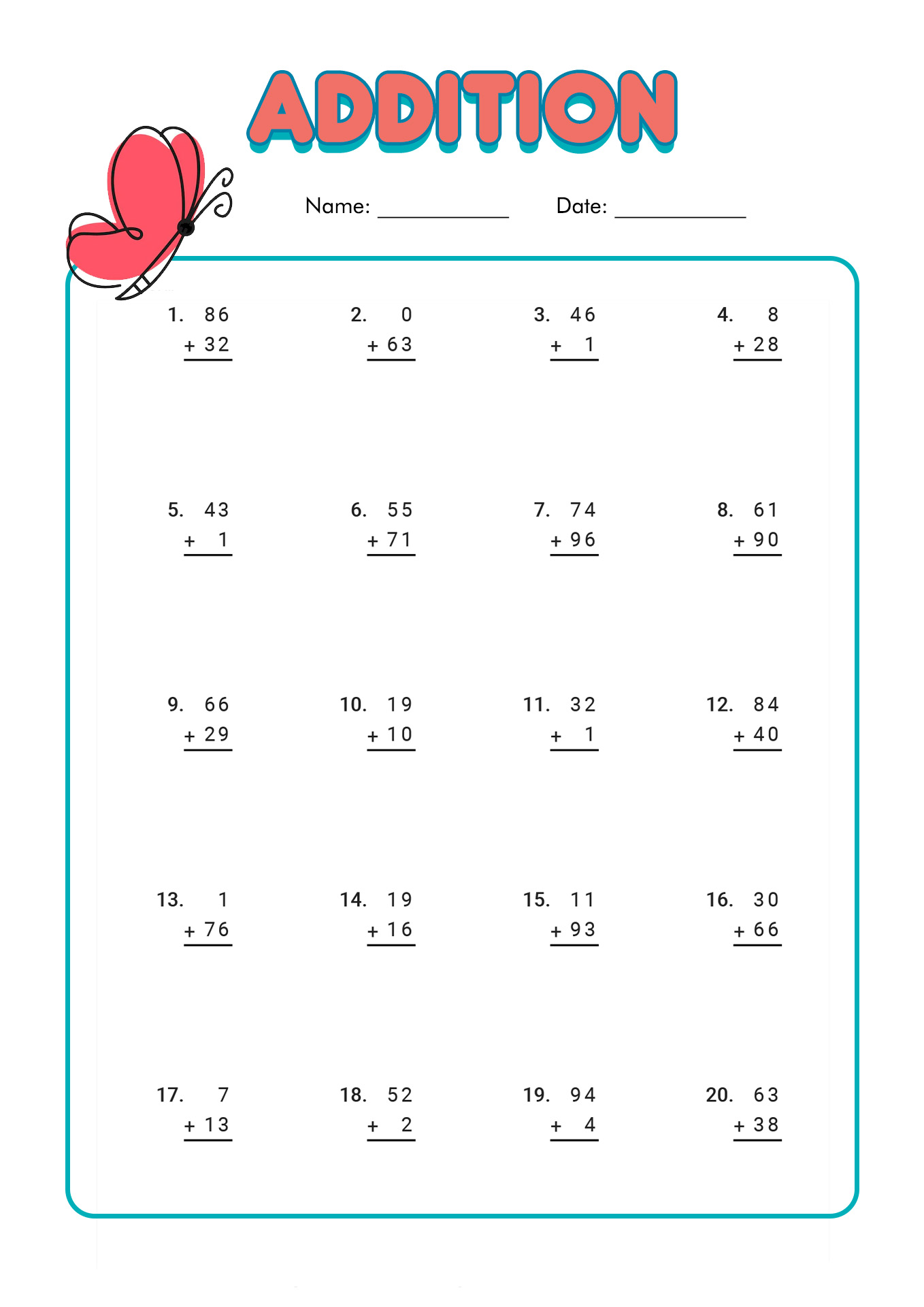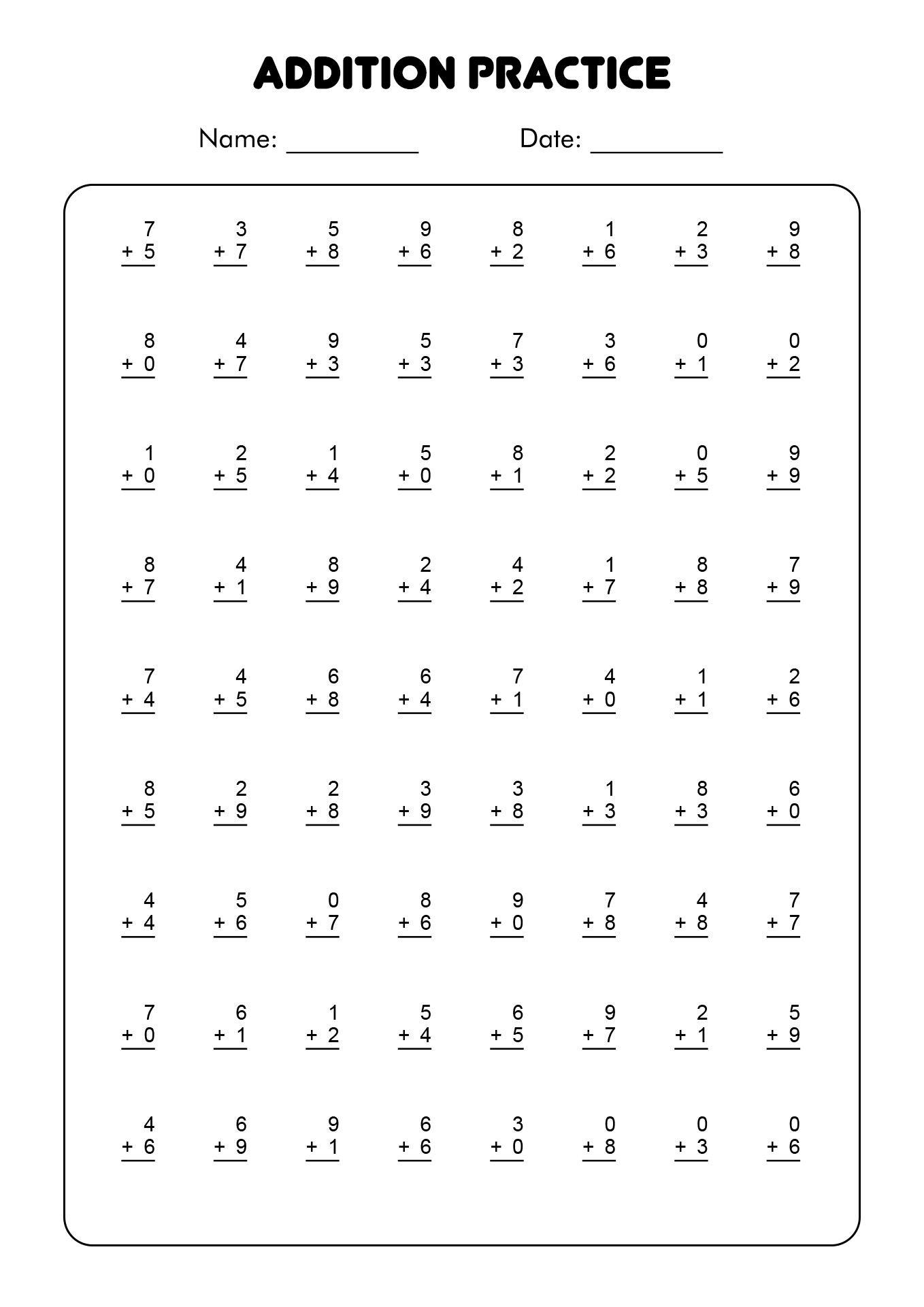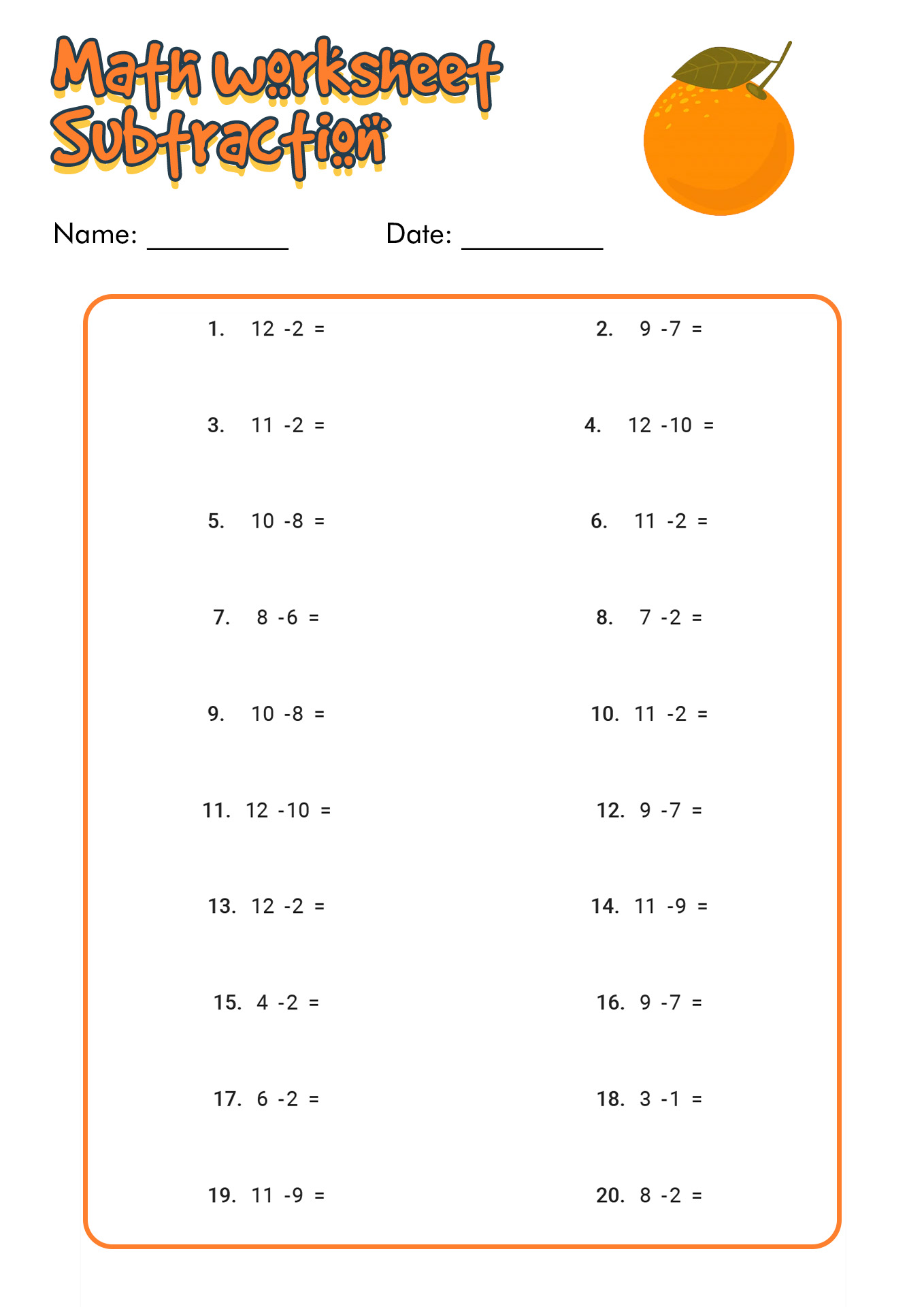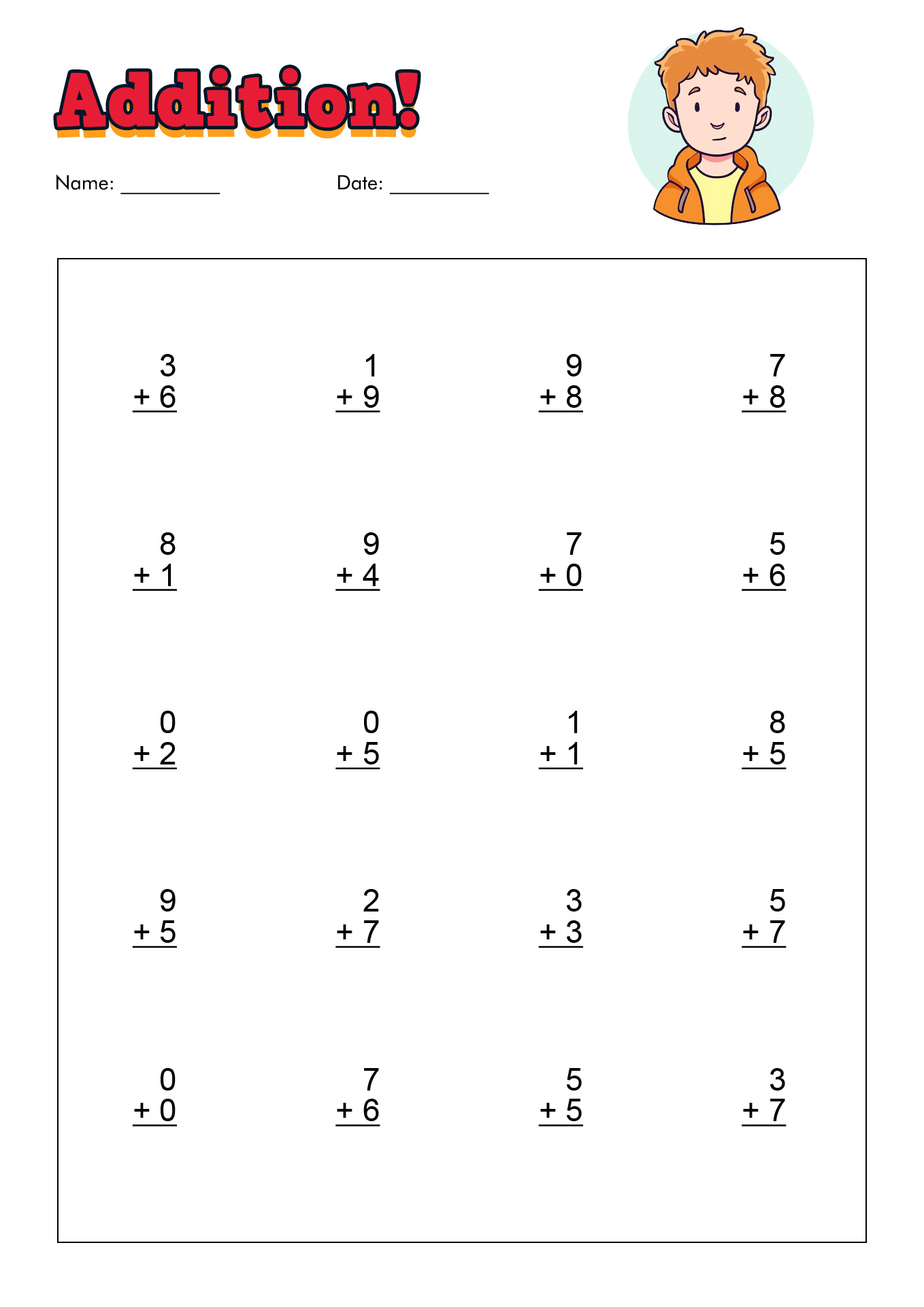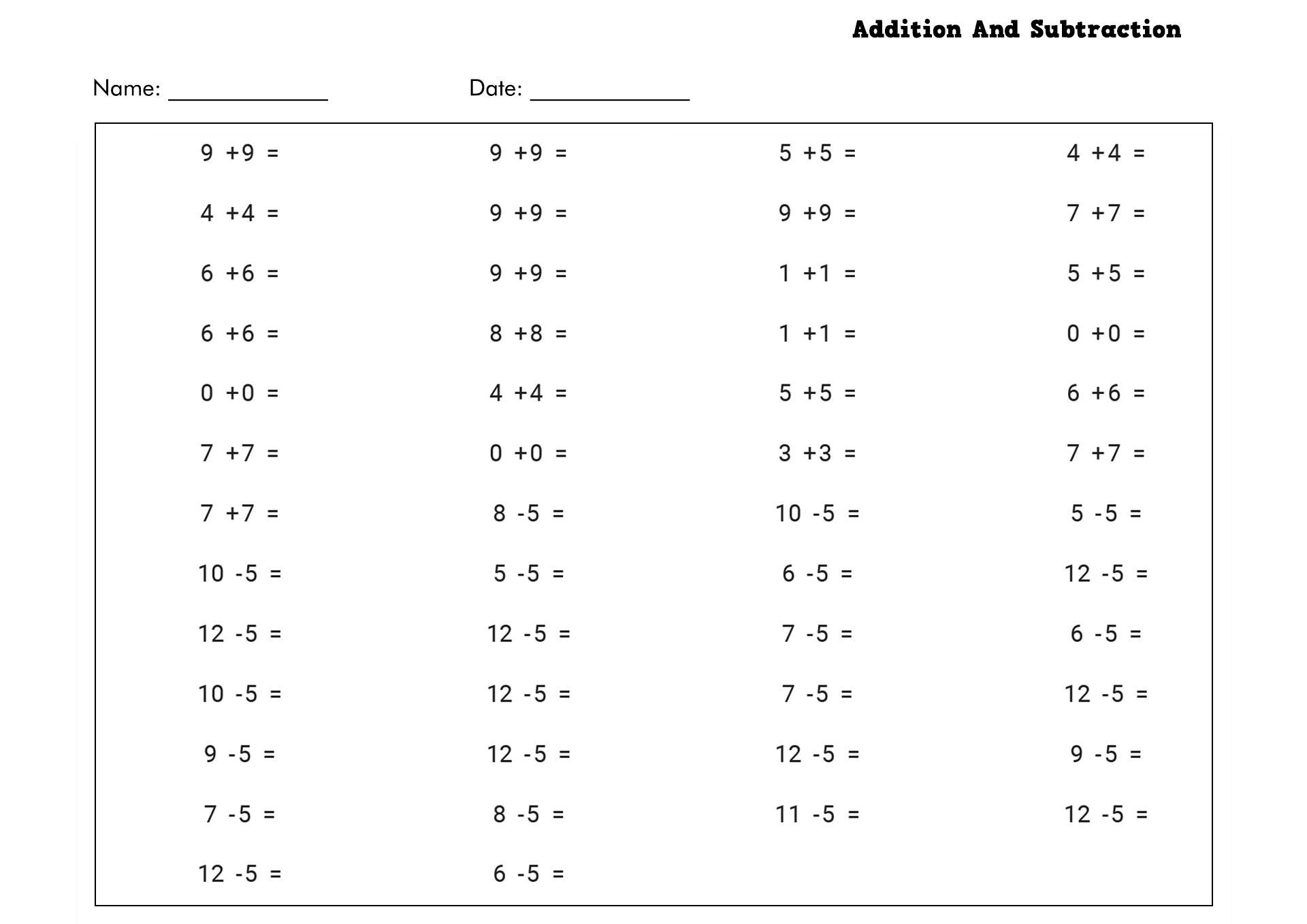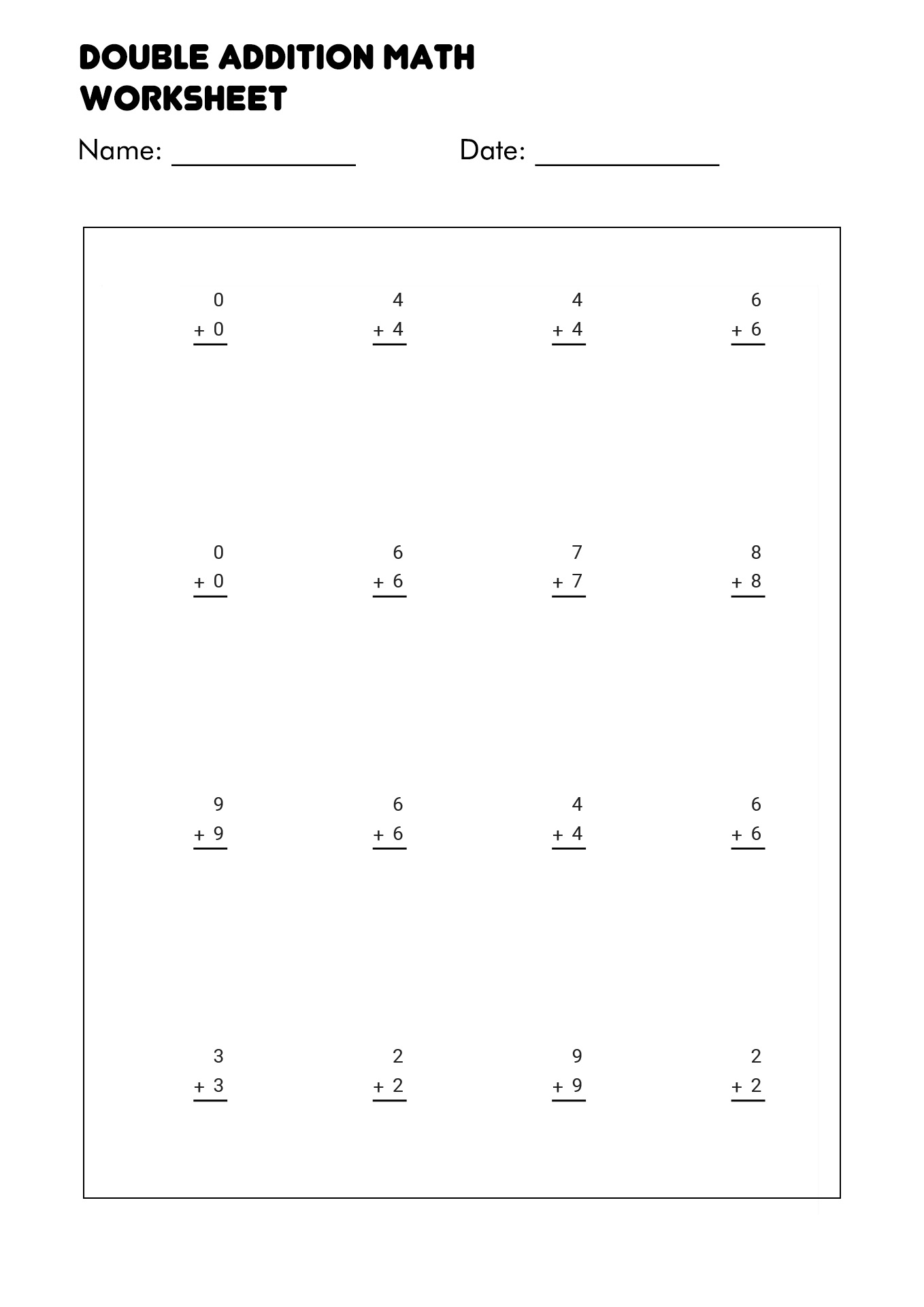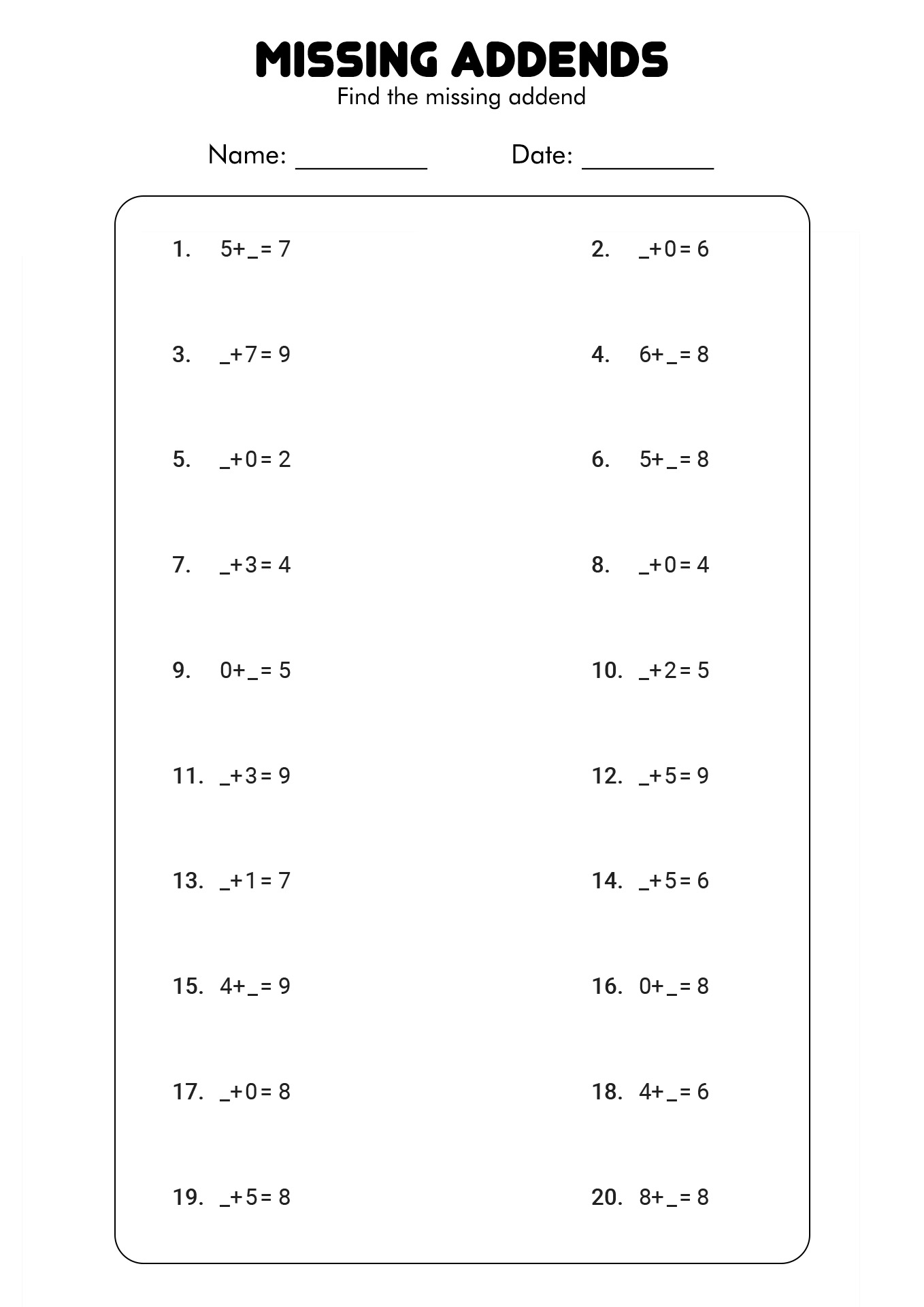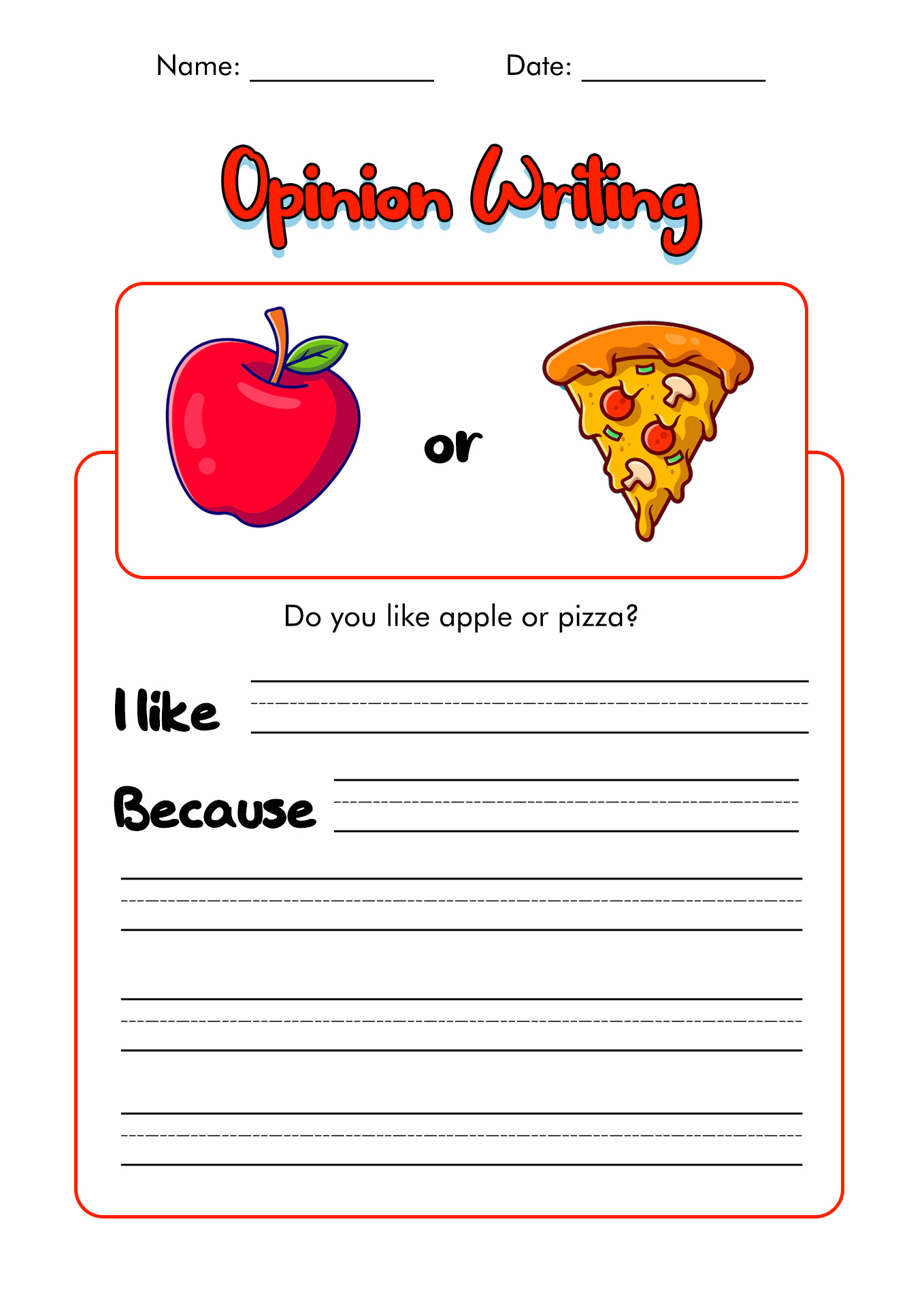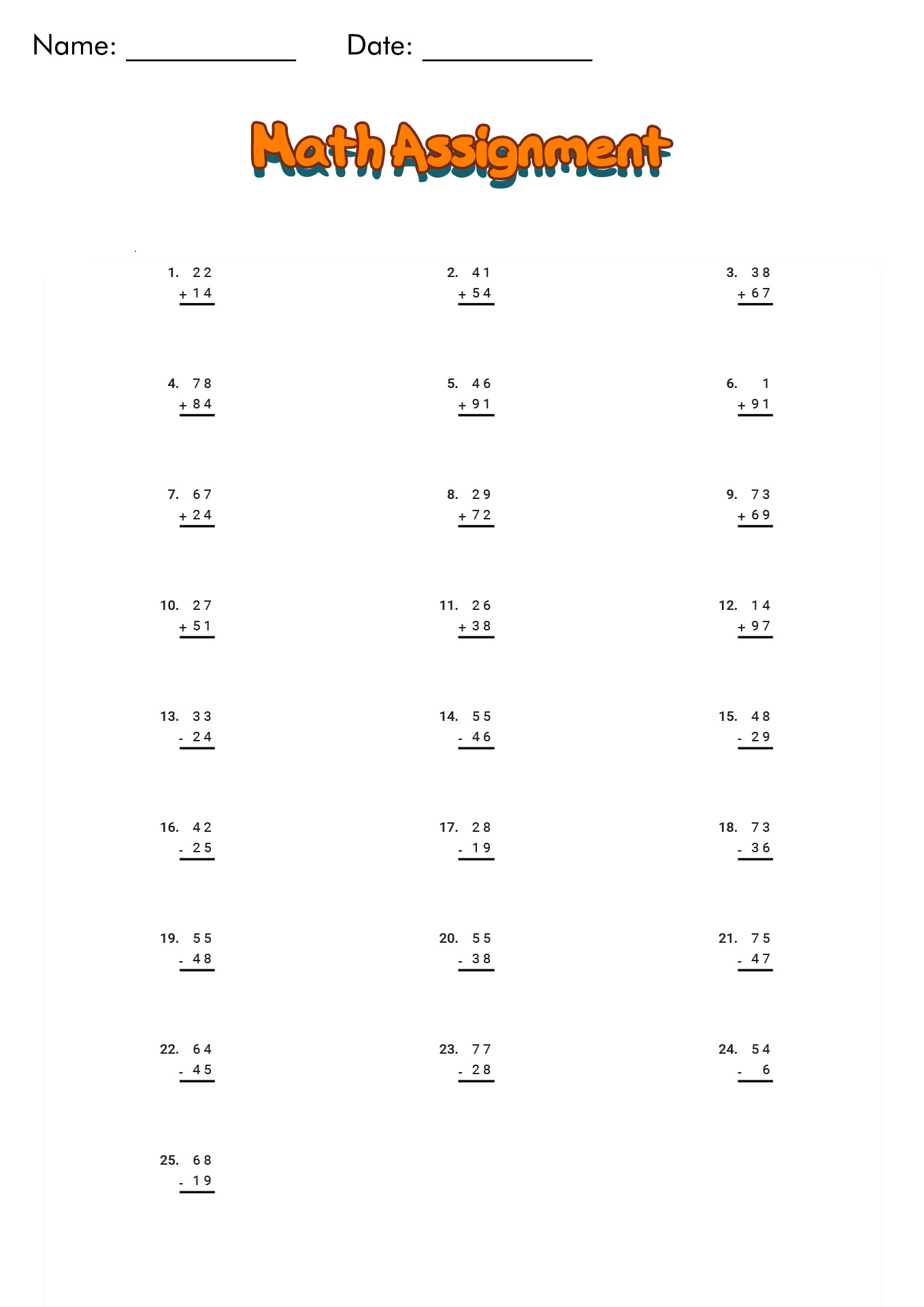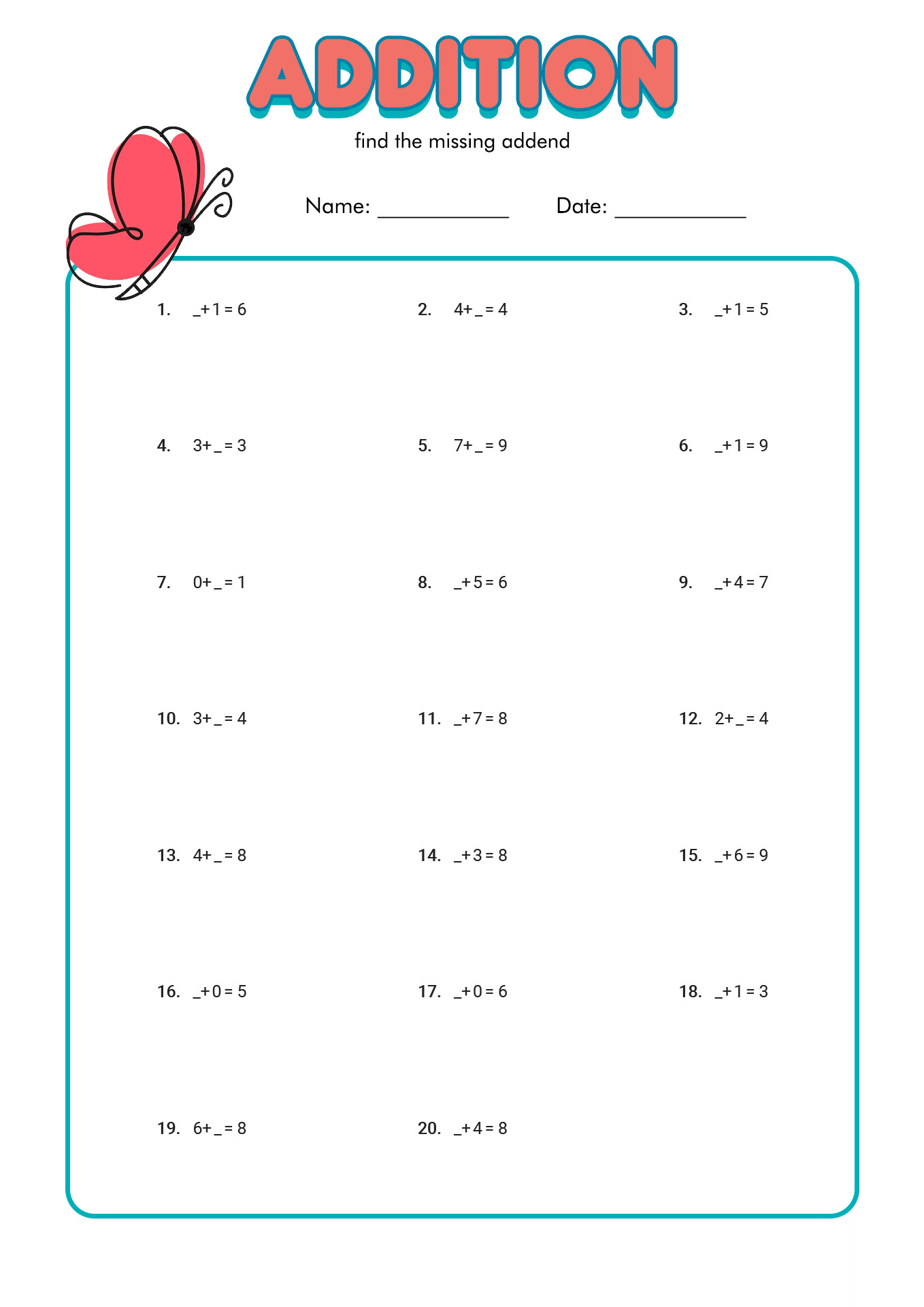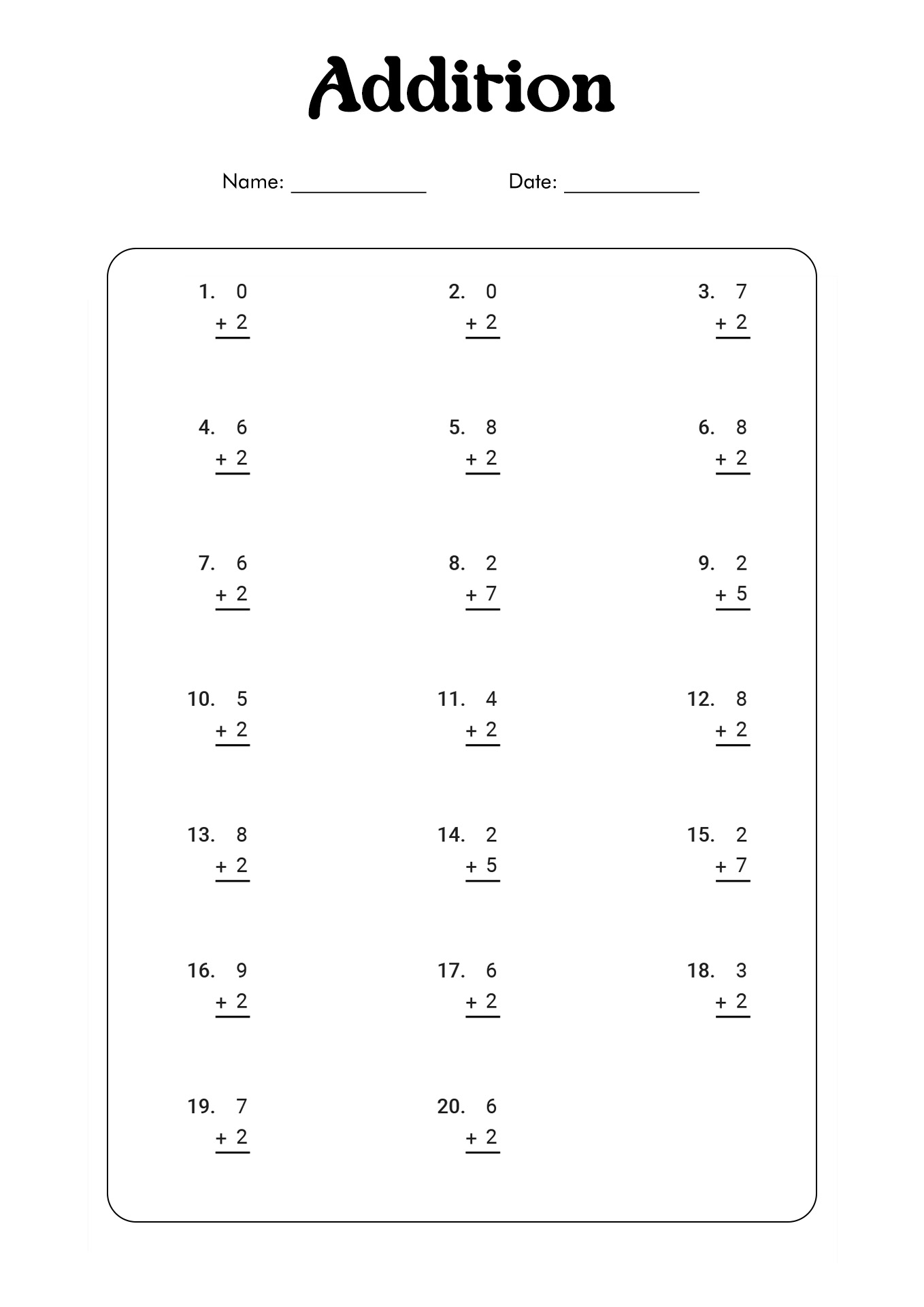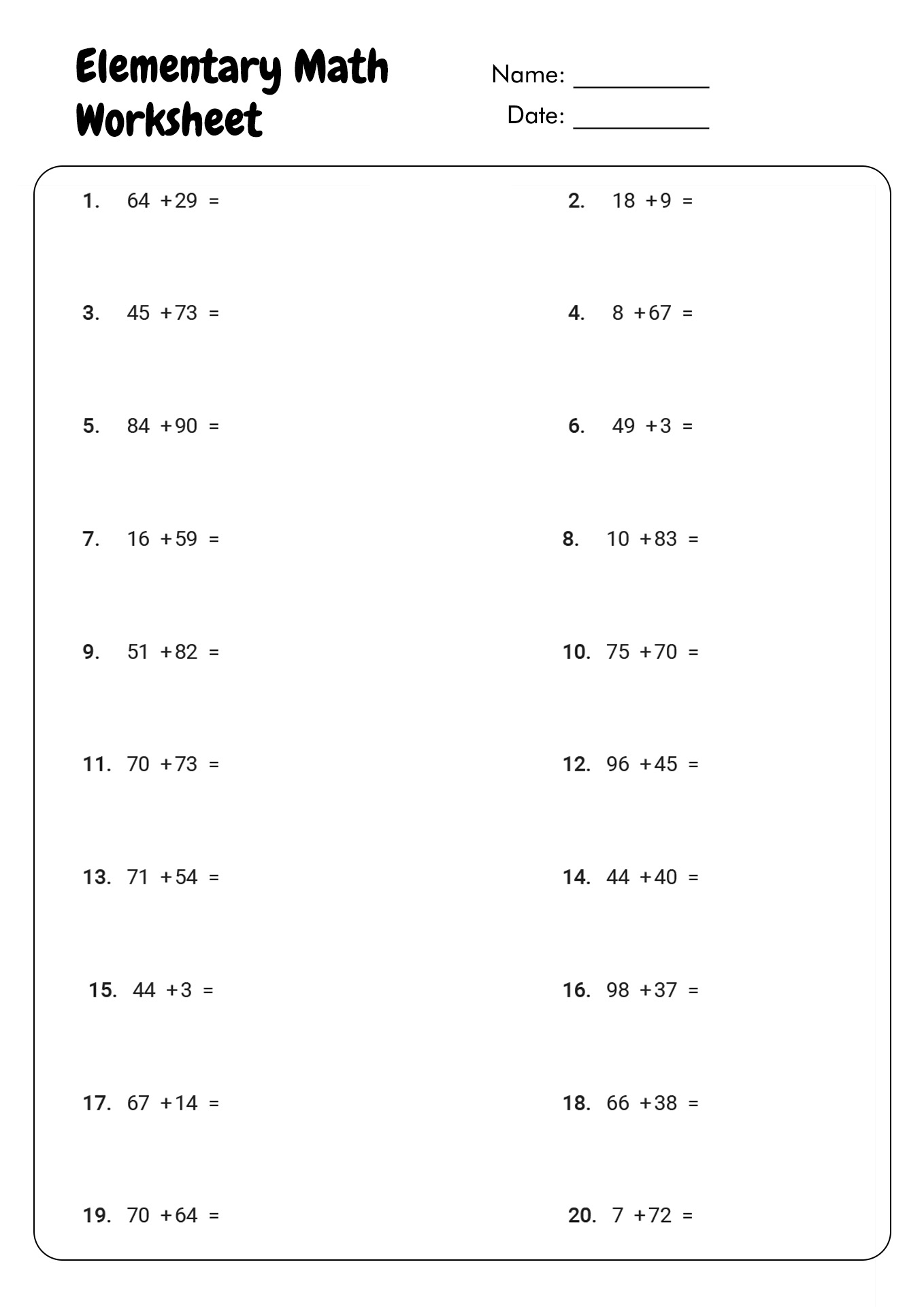Summary: Addition is one of the earliest mathematic operations that parents or teachers teach their children and students. Young learners need to master this knowledge because it is the foundation for acquiring further operations, especially multiplication. Mastering addition also can be used for complex mathematics, such as geometry, calculus, algebra and more. Parents or teachers can use objects to visualize the operations for the early introduction. For example, use candies or toys to represent the numbers. The children would not feel burdened over the numbers and symbols through this learning.

### What Does it Mean by Basic Arithmetic Operations?

Mathematics is one of the universal studies that every student should learn in every country. The rules for it are the same all over the world. It is because mathematics is a knowledge that transcends beyond language. People should master mathematics when they are young because it is the foundation of many daily-life activities. Understanding this knowledge will help someone to solve various problems in their life.

One of the matters that people should master regarding mathematics is arithmetic operations. Some experts agree that it is a simple measure of numbers in mathematics that deals with four types of functions. Addition, subtraction, multiplication and division are the four branches of the basic arithmetic operations. Each operation has its own designated universal symbols, which are + (addition), - (subtraction), x (multiplication) and ÷ (division). Young students must master these basic arithmetic operations because this knowledge will be the foundation of their future learning.

### How to Explain Addition in Mathematics to Young Students?

The addition is one of the earliest mathematic operations that parents or teachers teach their children and students. Young learners need to master this knowledge because it is the foundation for acquiring further operations, especially multiplication. Mastering addition also can be used for complex mathematics, such as geometry, calculus, algebra and more. It is also necessary knowledge for their daily life activities. According to Herbert Ginsburg from Columbia University, the purpose of the addition operation is to find out the sum of two or more quantities (numbers). In addition, the number we work with is "addends", and the result is "sum". To explain it better for young learners, we could say that addition means putting two or more numbers to find the total amount.

Parents or teachers can use objects to visualize the operations for the early introduction. For example, use candies or toys to represent the numbers. The children would not feel burdened over the numbers and symbols through this learning. Slowly, parents or teachers can move with simple practice through colourful and interesting worksheets for the children. The children must figure out the symbol of addition, the plus (+). Below is a list of concepts of addition that young students should master:

• The order of the number does not matter in additional operations.
• The opposite of addition is subtraction.
• The symbol of addition is the plus (+).

### Who Discovered Addition in Mathematics?

Addition is the oldest basic arithmetic operation, as our ancestors start to learn 'mathematics' through counting. In the early days, they did not use any numbers or symbols of addition because they counted the objects manually. The researchers found that Egyptians and Babylonians used addition around 2000 B. C. However, other sources also mentioned that the Chinese invented the addition operation. Meanwhile, Michael Stiple found the addition symbol (+) in the 16th century.

### What are the Properties of Addition in Mathematics?

As one of the four basic arithmetic operations, addition has rules. There are some rules, and they are known as properties. The purpose of the properties is to make the operation easier to study for the students. Below is the explanation of the four properties that students should understand:

• Commutative Property: The sum (results) of the addition operation would stay the same, even though the order of the addends (numbers) changes. For example, results of 4 + 7 and 7+4 are the same, 11.
• Associative Property: The different grouping patterns of the operations of three numbers would not change the results. For example, results of 3 + (5+4) and (3 +5) +4 are the same, 12.
• Distributive Property: The results of two quantities multiplied by the third number are the same as the total when each is multiplied by the third quantity. For example, results of 5 x (3+2) and 5 x 3 + 5 x 2 are the same, 25.
• Additive or Identity Property: The result of the addition operation between any number and zero (0) is the number itself. For example, 5 + 0 = 5.

### Why Do Young Learners Struggle in Learning Addition?

Learning addition is one of the things that students should master at a young age. It is understandable since addition holds an essential role in students' understatement of mathematics. However, introducing the concept of addition to young learners is not easy. The struggle comes from the lack of conceptual understanding of the students. Some students also have not mastered number recognition which makes them have difficulties in learning addition. The lack of learning motivation also could be the reason why students cannot grasp the concept of addition operation learning.

The information, names, images and video detail mentioned are the property of their respective owners & source.

### Popular Categories

Have something to tell us about the gallery?

Submit